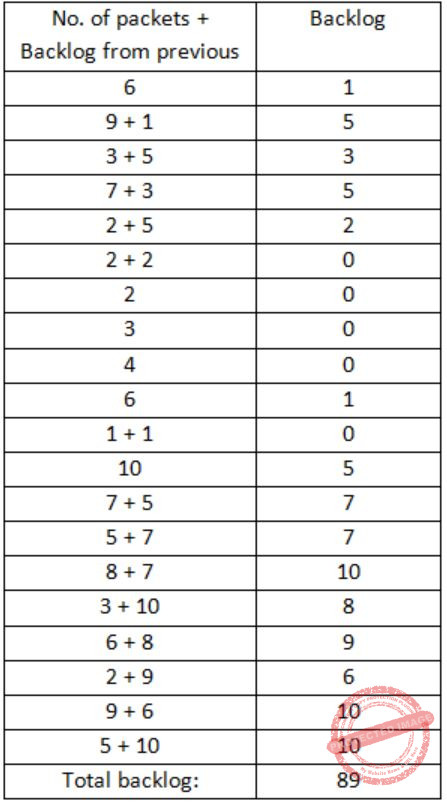## Access-Control-Methods

 Question 1

Let us consider a statistical time division multiplexing of packets. The number of sources is 10. In a time unit, a source transmits a packet of 1000 bits. The number of sources sending data for the first 20 time units is 6, 9, 3, 7, 2, 2, 2, 3, 4, 6, 1, 10, 7, 5, 8, 3, 6, 2, 9, 5 respectively. The output capacity of multiplexer is 5000 bits per time unit. Then the average number of backlogged of packets per time unit during the given period is

 A 5 B 4.45 C 3.45 D 0
Computer-Networks       Access-Control-Methods       GATE 2007-IT
Question 1 Explanation:
The capacity of multiplexer is 5000 bits per time unit. This means there are 5 packets per unit time since each source transmits a packet of 1000 bits in a unit time.
If the no. of packets transmitted is larger than 5 then the extra packets are backlogged. This means gets added to the next number and further backlog is calculated.Average no. of backlogged packets = 89/20 = 4.45
 Question 2

A broadcast channel has 10 nodes and total capacity of 10 Mbps. It uses polling for medium access. Once a node finishes transmission, there is a polling delay of 80 μs to poll the next node. Whenever a node is polled, it is allowed to transmit a maximum of 1000 bytes. The maximum throughput of the broadcast channel is

 A 1 Mbps B 100/11 Mbps C 10 Mbps D 100 Mbps
Computer-Networks       Access-Control-Methods       GATE 2007-IT
Question 2 Explanation:
Efficiency = Transmission time(Tt)/Transmission time+Polling delay
(Tt) = 1000bytes/10×106bits/sec = 800μs
Polling delay = 80 μs
Efficiency = 800/800+80 = 800/880 = 10/11
Maximum throughput is
= 10/11 × 10 Mbps
= 100/11 Mbps
 Question 3
In a token ring network the transmission speed is 107 bps and the propagation speed is 200 meters/ μs. The 1-bit delay in this network is equivalent to:
 A 500 meters of cable B 200 meters of cable C 20 meters of cable D 50 meters of cable
Computer-Networks       Access-Control-Methods       ISRO-2016       Video-Explanation
Question 3 Explanation:
Tt = L / B => 1/ 107 = 0.1 microsec
Given Tp = 200 m / microsec
In, 1 microsec it covers 200m
Therefore, in 0.1 microsec it is 200 * 0.1 = 20 meters
 Question 4

A slotted ALOHA network transmits 200-bit frames using a shared channel with a 200 Kbps bandwidth. Find the throughput of the system, if the system (all stations put together) produces 250 frames per second :

 A 49 B 368 C 149 D 151
Computer-Networks       Access-Control-Methods       UGC-NET CS 2018 JUNE Paper-2
Question 4 Explanation:
Step-1:
Given data,
Slotted ALOHA transmits = 200 bit frames
Bandwidth = 200 Kbps
System produces = 250 frames per second
Throughput = ?
Step-2:
Pure ALOHA formula S = G * e-2G
Slotted ALOHA formula S = G * e-G
Frame transmission time = 200/200 kbps = 1ms
Here,
G = ¼ and S = G * e-G
= 0.195 (or) 19.5%
System produces = 250 * 0.195
= 48.75
There are 4 questions to complete.

Register Now